# 云环境下集合隐私计算-解读

## 基础

### 哥德尔编码([a_1,x_2,...,x_m])称作有穷序列((a_1,x_2,...,x_m))的哥德尔数。

### 同态加密算法

#### ElGamal

1、加密结构：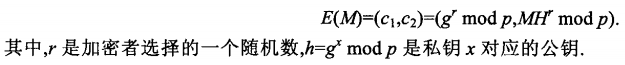2、乘法计算：#### Paillier

1、加密结构2、加法计算## 保密计算求集合并集方案

### 原理

#### 1-r编码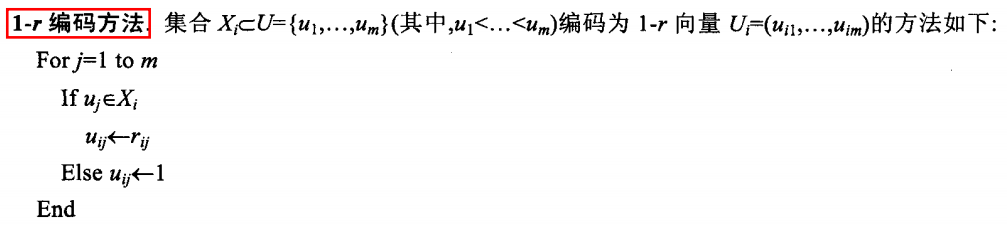#### 举例### 具体方案1

（1）云服务器(P_n)将自己的公钥(pk)与参数(params)公开，并保留私钥(sk)（2）每个云服务器(P_i(i=1,...,n))计算：

• 将自己的秘密集合(X_i)编码为向量(U_i)
(X_i)使用1-r编码方法编码为向量(U_i=(u_{i,1},...,u_{i,m}))

• (P_n)的公钥(pk)与参数(params)加密(U_i)(E(U_i))
将向量(U_i)中的每个分量分别加密，即(E(U_i)=(E(u_{i,1}),...,E(u_{i,m})))

• 将密文向量(E(U_i))随机分成(k_i)份并发送给(n)个云服务器中的(k_i)
为了将数据混淆来保证(U_i)的安全，每台服务器需要将(E(u_{i,j}),j=1,...,m)分为(k_i(k_i leqslant n))(E(u_{i,j})_1,...,E(u_{i,j})_{k_i})，（(k_i)其他服务器是不知道的），需要满足:(E(u_{i,j})_1...E(u_{i,j})_{k_i}=E(u_{i,j}))
(P_i)每次从每个密文分量(E(u_{i,j})_1,...,E(u_{i,j})_{k_i})中选取一个，共选取(k_i)次，构成(U_i)(k_i)份密文(E(U_i)_1,...,E(U_i)_{k_i})，每份（向量）里面(m)个元素。
如果直接对(E(u_{i,j}),j=1,...,m)进行分解因子，计算量大，所以这里使用模运算进行分解因子，具体如下：
选取(k_i)个随机数(r_{i,1},..,r_{i,k_i}in Z_p^*)，需要满足(r_{i,1}....r_{i,k_i}=1 mod p)，从(k_i)个随机数中随机选择一个，如(r_{i,1})，和(E(u_{i,j}))相乘，即(E(u_{i,j})_1=E(u_{i,j}).r_{i,1})，然后将(E(u_{i,j})_2,...,E(u_{i,j})_{k_i})(r_{i,2},...,r_{i,k_i})表示，进而就能将(E(U_i))"拆分"(k_i)份，且满足：(E(u_{i,j})_1...E(u_{i,j})_{k_i}=r_{i,1}.E(u_{i,j}).r_{i,2}...r_{i,k_i}=E(u_i,j))
最后将这个(k_i)个密文向量(E(U_i)_1,...,E(U_i)_{k_i})发送给k个云服务器（可以包括自己）。

• 把收到的所有密文向量对应的分量相乘得到新的密文向量(E(U_i'))，并发送给(P_n)
（3）云服务器(P_n)计算：

• 所有收到的密文向量对应的分量相乘，得到(E(U'))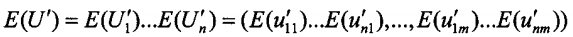由于每个服务器都是半诚实的，不会加入额外的信息，所以(P_n)构造的密文向量(E(U'))是所有参与者密文向量各个分量的乘积，即:• 用自己的私钥(sk)解密(E(U'))得到(U')

[U'=(u_1',...,u_m') ]

• 根据(U')中不为1的分量得到((sigma _1,...,sigma _h))
• 公布((sigma _1,...,sigma _h))

### 举例

（1）每台服务器(P_i)编码各自的集合数据(X_i)(U_i)；然后再使用公钥(pk)加密，即(E(U_1),E(U_2),E(U_3),E(U_4))；再将其拆分为(k)份，分别发送给其他两个服务器（自己留一份）
（2）在发送完后，(P_i)就有可能收到其他服务器发来的密文向量，然后将每份的密文向量对应的元素相乘得到(E(U_i'))，发送给(P_4)
（3）(P_4)得到：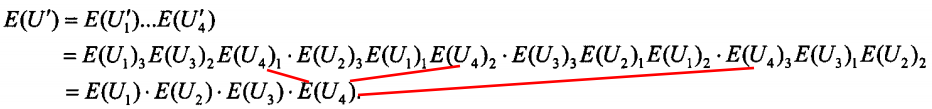（4）(P_4)(E(U'))解密，根据(U')中不为1的元素得到并集((sigma _1 ,...,sigma _h ))## 保密计算求集合并集优化方案

### 基本思想

#### 0-r编码+哥德尔编码

（1）总集合(U=(u_1,...,u_m),(u_1<....<u_m))对应集合(P=(p_1,...,p_m),(p_1,...,p_m))是不等的互素数
（2）对于集合(X_iin U)，将其按照0-r编码方式编码为向量(U_i=(u_{i,1},...,u_{i,m}))，具体是，若(u_jin X_i)，则(u_{i,j}=r_{i,j})，其中(r_{i,j}in [1,m])是一个随机数且(r_{i,j}neq 0)，否则(u_{i,j}=0)，然后借助集合(P)利用哥德尔编码将(U_i)编码为一个自然数(x_i)，即：#### 举例### 具体方案2

（1）云服务器(P_n)保留私钥(sk)，公布公钥(pk)和系统参数(params)（2）每个云服务器(P_i)计算如下：

• 根据上面改造的编码方法将自己的秘密集合(X_i)编码为自然数(x_i)

• 用公钥(pk)加密(x_i)(E(x_i))
直接发送(E(x_i))是不安全的，因为(P_n)可以直接解密，所以需要混淆密文与云服务器的对应关系！

• (E(x_i))随机分为(k_i)份并发送给(k_i)个服务器• 将收到的所有密文相乘得到新的密文(E(x_i'))，并发送给(P_n)（3）云服务器(P_n)计算如下：

• 把所有收到的密文相乘，得到(E(x))
• 用私钥(sk)解密(E(x))得到(x)
• (x)用算术基本定理展开得到向量(U')
• 根据(U')中不为0的分量得到集合((sigma _1,...,sigma _h))### 云计算下的改造方案（1）用户自己编码，然后拆分混淆，发给服务器

## 保密计算求集合交集方案

### 协议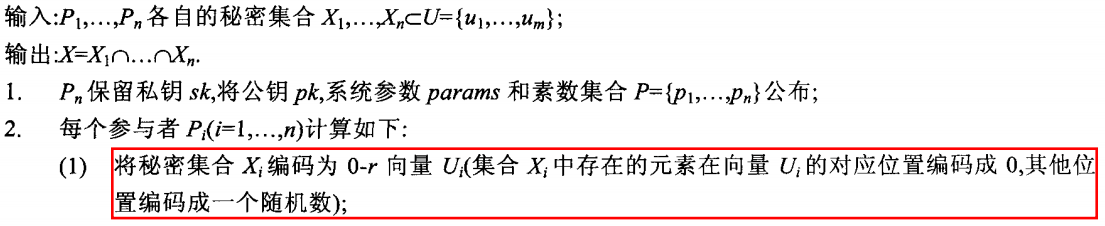### 举例

（1）集合(U=(101,102,103,104,105,106,107,108,109,110))对应集合(P=(2,3,5,7,11,13,17,19,23,31))，假设要计算3个集合(X_1=(101,105,107),X_2=(103,105,108),X_3=(105,106,109))的交集，根据0-r编码将其编码为向量(U_1,U_2,U_3)，即：

[U_1=(0,1,2,3,0,4,0,5,6,7),U_2=(1,2,0,4,0,5,6,0,7,8),U_3=(1,2,3,4,0,0,7,8,0,9) ]

（2）将向量(U_1,U_2,U_3)借助集合(P)利用哥德尔编码编码为自然数(x_1,x_2,x_3)，即：

[x_1=[0,1,2,3,0,4,0,5,6,7]=3^1.5^2.7^3.13^4.19^5.23^6.31^7;x_2=[1,2,0,4,0,5,6,0,7,8]=2^1.3^2.7^4.13^5.17^6.23^7.31^8;x_3=[1,2,3,4,0,0,7,8,0,9]=2^1.3^2.5^3.7^4.17^7.19^8.31^9 ]

（3）将这三个自然数相乘得到：

[x=x_1.x_2.x_3=2^2.3^5.5^5.7^{11}.11^0.13^9.17^{13}.19^{13}.21^{13}.31^{24} ]

（4）即得到向量(U'=(2,5,5,11,0,9,13,13,13,24))，根据向量(U')中为0的分量得到交集：

[X_1 cap X_2 cap X_3=(105) ]